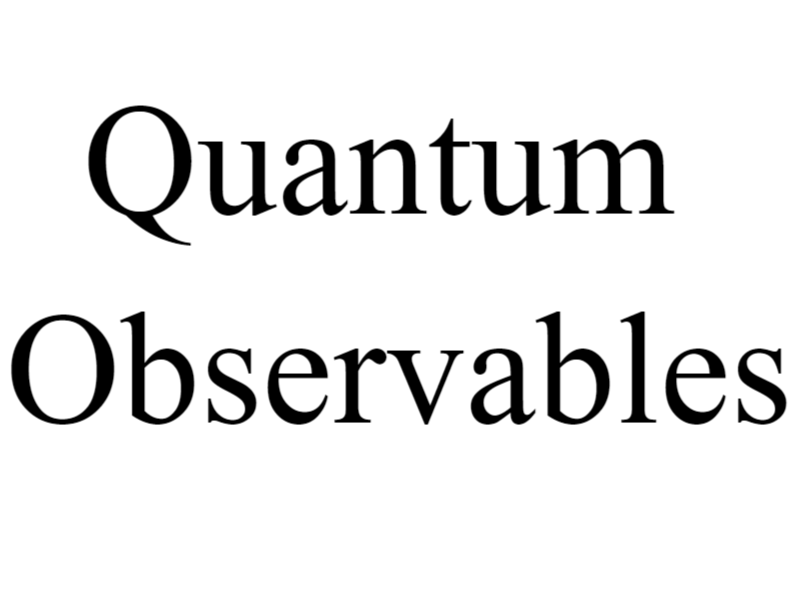### Quantum Observables: Properties, Measurements, and Physical Significance

Abstract:

Quantum observables play a central role in quantum mechanics, representing physical quantities that can be measured in quantum systems. This paper provides a comprehensive exploration of quantum observables, their mathematical representation, measurement processes, and physical significance. We discuss the properties and characteristics of quantum observables, their connection to measurement operators, and their relevance in understanding the behavior and properties of quantum systems.

Keywords: Quantum Observables, Quantum Mechanics, Measurement, Measurement Operators, Probability Distributions.

Introduction:

Quantum observables are fundamental concepts in quantum mechanics, representing physical quantities that can be measured in quantum systems. They provide a bridge between the mathematical formalism of quantum mechanics and observable phenomena. This paper delves into the nature and properties of quantum observables, their mathematical representation, measurement processes, and their significance in understanding the behavior and properties of quantum systems.

Mathematical Representation of Quantum Observables:

Quantum observables are mathematically represented by Hermitian operators, known as measurement operators or observables. These operators act on the Hilbert space of the quantum system, and their eigenvalues correspond to the possible outcomes of measurements. The eigenvectors associated with the eigenvalues define the basis states in which the system can be found after a measurement.

Properties and Characteristics of Quantum Observables:

Quantum observables possess several key properties and characteristics. They are represented by Hermitian operators, guaranteeing real eigenvalues and orthogonal eigenvectors. Observables can have discrete or continuous spectra, depending on the nature of the physical quantity they represent. They are subject to the uncertainty principle, which places limits on the simultaneous measurement of certain pairs of observables.

Measurement Processes and Probability Distributions:

When a quantum observable is measured, the system undergoes a state change, collapsing into one of the eigenstates of the observable. The probability of obtaining a particular measurement outcome is determined by the squared modulus of the projection of the system’s initial state onto the corresponding eigenstate. The measurement process is probabilistic due to the inherent nature of quantum mechanics.

Connection to Measurement Operators:

Quantum observables are intimately connected to measurement operators, which mathematically represent the observables. Each observable corresponds to a Hermitian operator, and the eigenvalues of the operator correspond to the possible outcomes of a measurement. The eigenvectors of the operator represent the states in which the system can be found after the measurement.

Physical Significance and Interpretation:

Quantum observables provide essential information about the properties, behavior, and dynamics of quantum systems. They allow for the calculation of expectation values, which represent the average values of observables in a given quantum state. Observables are fundamental in describing the evolution of quantum systems, characterizing quantum states, and predicting measurement outcomes.

Applications and Future Perspectives:

Quantum observables have diverse applications in quantum mechanics and its applications, such as quantum information processing, quantum cryptography, and quantum metrology. The understanding and manipulation of observables are crucial for the development of quantum technologies and the exploration of quantum phenomena. Ongoing research focuses on the development of novel measurement techniques, the study of entangled observables, and the role of observables in quantum control.

Conclusion:

Quantum observables are essential components in quantum mechanics, representing physical quantities that can be measured in quantum systems. Their mathematical representation, measurement processes, and physical significance enable us to explore and understand the behavior and properties of quantum systems. The study of quantum observables has far-reaching implications in various fields, paving the way for the development of quantum technologies and the advancement of our understanding of the quantum world.

References:

1. Sakurai, J. J., & Napolitano, J. (2017). Modern Quantum Mechanics. Cambridge University Press.
2. Cohen-Tannoudji, C., Diu, B., & Laloe, F. (2006). Quantum Mechanics: Concepts and Applications. John Wiley & Sons.
3. Nielsen, M. A., & Chuang, I. L. (2010). Quantum Computation and Quantum Information. Cambridge University Press.
4. Ballentine, L. E. (1998). Quantum Mechanics: A Modern Development. World Scientific.
5. Bransden, B. H., & Joachain, C. J. (2000). Quantum Mechanics. Pearson Education.